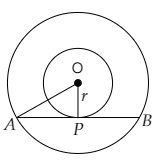# Two concentric circles are of radii 5 cm and 3 cm. Find the length of the chord of the larger circle which touches the smaller circle.

Given:

Two concentric circles are of radii $5 \mathrm{~cm}$ and $3 \mathrm{~cm}$.

To do:

We have to find the length of the chord of the larger circle which touches the smaller circle.

Solution:Let $R$ be the radius of outer circle and $r$ be the radius of small circle of two concentric circles.
$AB$ is the chord of the outer circle and touches the smaller circle at $P$
Join $OP$ and $OA$.

This implies,

$OP\ \perp\ AB$ and bisects it at $P$.

$OA=R$ and $OP=r$

$OA=5\ cm$

$OP=3\ cm$

In right angled triangle $OAP$,

$AP=\sqrt{OA^{2}-OP^{2}}$

$=\sqrt{5^{2}-3^{2}}$

$=\sqrt{25-9}$

$=\sqrt{16}$

$=4\ cm$

$AB=2AP$

$=2 \times 4\ cm$

$=8\ cm$

The length of the chord of the larger circle is 8 cm.

Updated on: 10-Oct-2022

61 Views